# Knowledge Base

## Solution 34599: Solving Simultaneous Equations on the TI-83 Plus and TI-84 Plus Family of Graphing Calculators.

### How can I solve simultaneous equations on the TI-83 Plus and TI-84 Plus family of graphing calculators?

Simultaneous equations can be solved by entering the coefficients of the equations in a matrix, and then using the rref() function on your matrix.

For Example:

Solve for x and y
3x + -2y = 12
6x + 4y = -3

Solution: First, begin by inputting the matrix. To do so, please follow the steps below:

1) Press [2nd] [MATRIX] to bring up the Matrix Menu.
2) Scroll to Edit, and press 1:[A].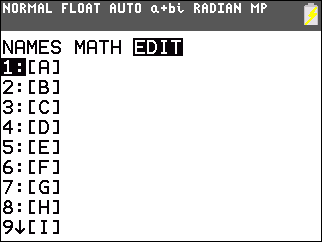3) Input the dimensions of your matrix by pressing  [ENTER]  [ENTER].
4) Input the matrix entries, pressing enter after each value. Your entries should be 3, -2, 12, 6, 4 and -3.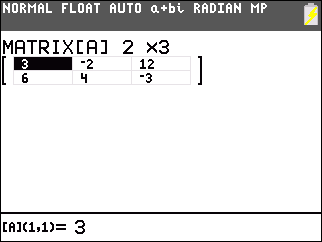5) Press [2nd] [QUIT] to exit out of the editing screen.

To solve your system of equations, you'll now need to use the rref function. To find the reduced row echelon form using the rref( function:

6) Press [2nd] [MATRIX].
7) Scroll to "MATH" by pressing the right arrow key one time.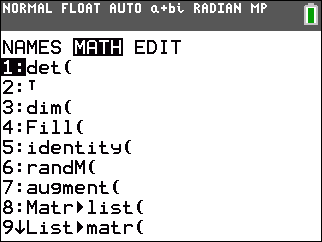8) Scroll down until you see "rref(", which is the function for reduced row echelon form. Press [ENTER] on that option, which then pastes the function onto your home screen.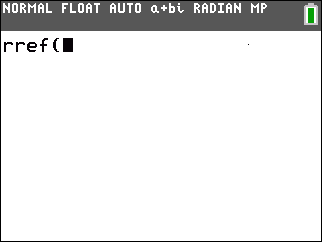9) Press [2nd] [MATRIX] [ENTER], which pastes Matrix A into the rref command.
10) Close the parenthesis by pressing [ )].
11) Press [ENTER] to display the answer.

The screen will show: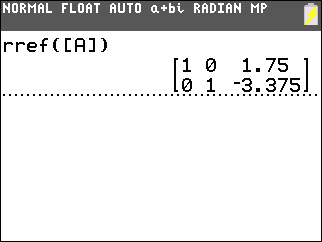Therefore the solution is x = 1.75 and y = -3.375

Please Note: In addition the Polynomial Root Finder and Simultaneous Equation Solver App can be downloaded onto the TI-84 Plus CE, TI-84 Plus C Silver Edition and TI-84 Plus and TI-83 Plus calculators to provide this feature.

Please see the TI-83 Plus and TI-84 Plus Family guidebooks for additional information.